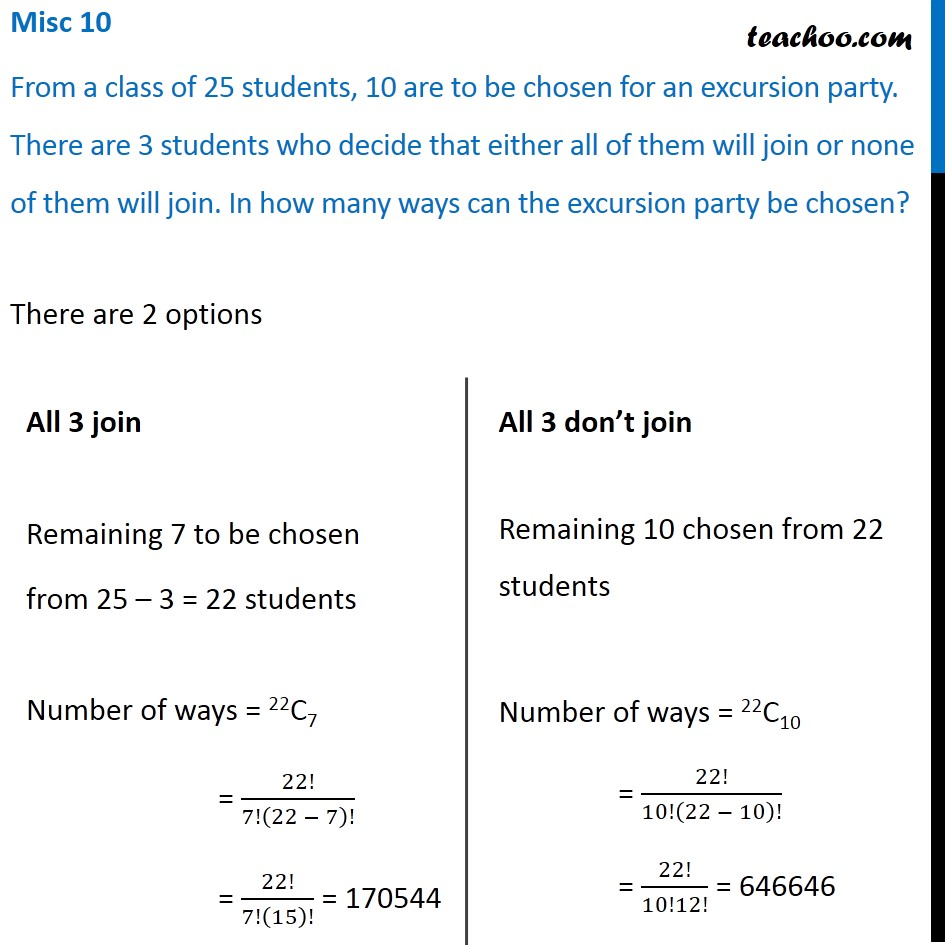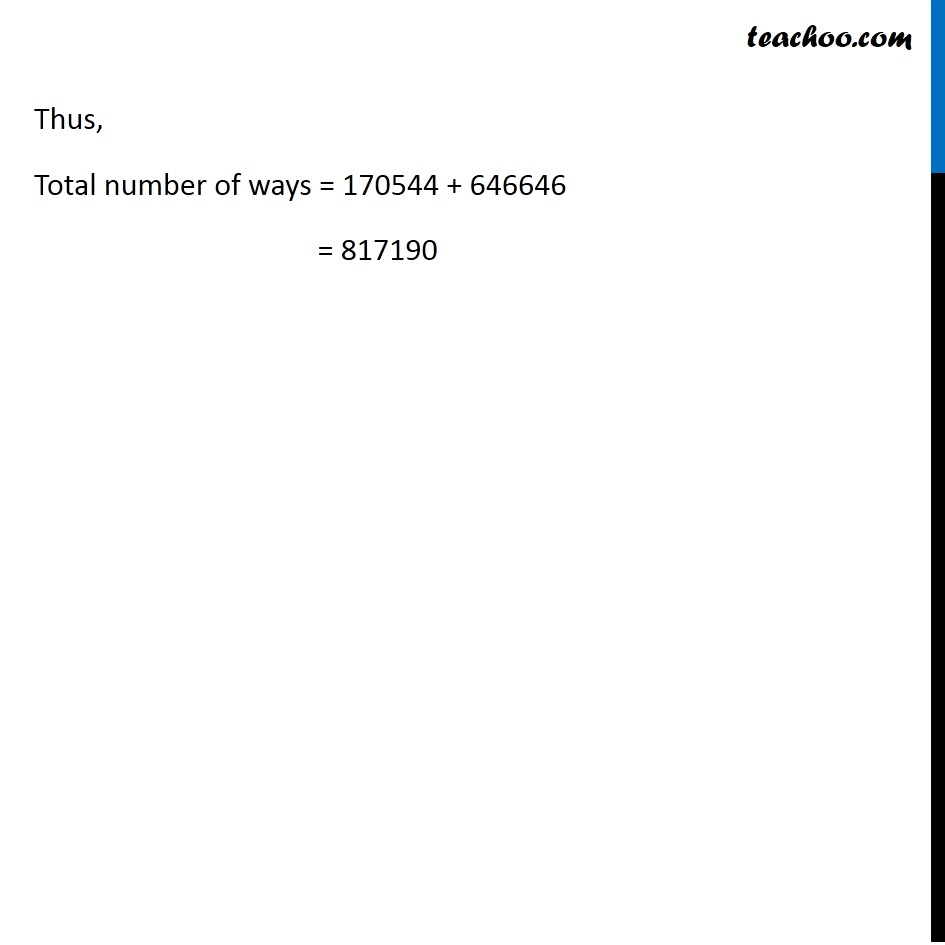1. Chapter 7 Class 11 Permutations and Combinations (Term 2)
2. Serial order wise
3. Miscellaneous

Transcript

Misc 10 From a class of 25 students, 10 are to be chosen for an excursion party. There are 3 students who decide that either all of them will join or none of them will join. In how many ways can the excursion party be chosen? There are 2 options All 3 join Remaining 7 to be chosen from 25 – 3 = 22 students Number of ways = 22C7 = 22!/7!(22 − 7)! = 22!/7!(15)! = 170544 All 3 don’t join Remaining 10 chosen from 22 students Number of ways = 22C10 = 22!/10!(22 − 10)! = 22!/10!12! = 646646 Thus, Total number of ways = 170544 + 646646 = 817190